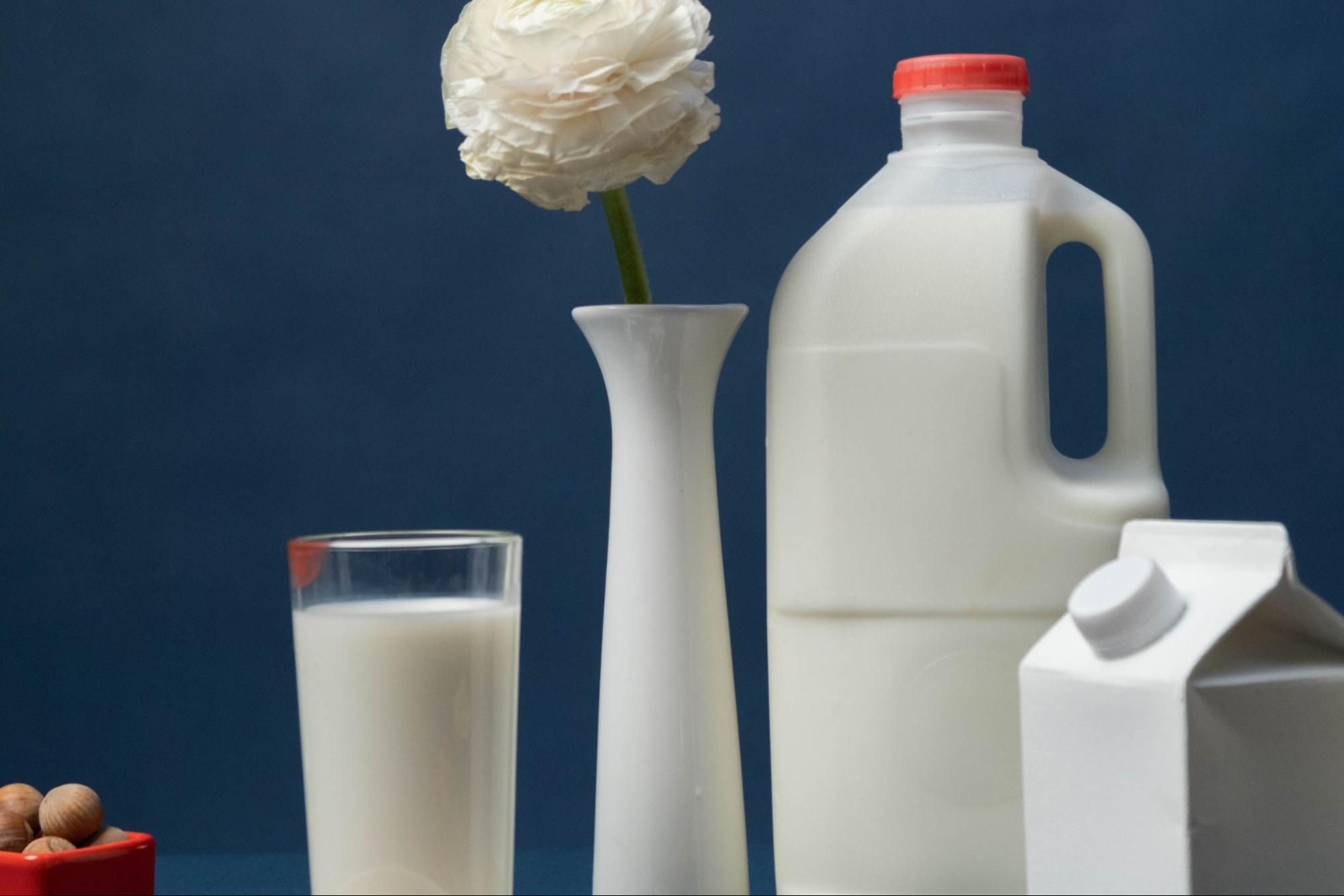# Find Out Now: How Many Gallons in a mL## How Many Gallons in a mL

If you’ve ever wondered how many gallons are in a milliliter, you’re not alone. The conversion between these two units of measurement can be a bit perplexing. As an expert, I’ll clear up any confusion and provide you with a straightforward answer.

To put it simply, there are 0.000264172 gallons in one milliliter. This means that if you have a certain volume measured in milliliters, you can convert it to gallons by multiplying the value by this conversion factor. Understanding this conversion can come in handy when dealing with different liquid measurements, especially if you’re working with international recipes or scientific calculations.

So whether you’re looking to convert milliliters to gallons for cooking purposes or for academic reasons, knowing this conversion ratio will save you time and ensure accuracy in your calculations. Stay tuned as I dive deeper into the topic and provide more insights on converting between different units of volume measurement.

## Understanding Milliliters and Gallons

Milliliters and gallons are two common units of measurement used to quantify volume. While milliliters are typically used in the metric system, gallons are a more familiar unit of measurement in the United States. Understanding the relationship between these two units can be helpful when converting measurements or comparing quantities.

1. What is a Milliliter? A milliliter (ml) is a unit of volume in the metric system. It is equal to one thousandth of a liter, which makes it a relatively small unit of measurement. To put it into perspective, imagine filling up an average-sized teaspoon with liquid; that would roughly be equivalent to 5 milliliters. Milliliters are commonly used for measuring smaller amounts of liquid, such as medication doses or recipe ingredients.
2. What is a Gallon? On the other hand, gallons are larger units of volume primarily used in the United States and some other countries that have adopted imperial measurements. There are different types of gallons, including US liquid gallons and UK imperial gallons, but for simplicity, we will focus on US liquid gallons here. One US liquid gallon is equivalent to approximately 3,785 milliliters.
3. Converting Between Milliliters and Gallons Converting between milliliters and gallons involves multiplying or dividing by conversion factors based on their relationship:
• To convert from milliliters to gallons: divide the number of milliliters by 3,785.
• To convert from gallons to milliliters: multiply the number of gallons by 3,785.

For example:

• If you have 500 ml and want to convert it to US liquid gallons: divide 500 by 3,785. The result is approximately 0.13 US liquid gallon.
• Conversely, if you have 2 US liquid gallons and want to convert it to milliliters: multiply 2 by 3,785. The result is approximately 7,570 ml.

In summary, milliliters and gallons are units of volume used to quantify liquids. Milliliters are smaller units commonly used in the metric system, while gallons are larger units primarily used in the United States. Converting between these two units requires multiplying or dividing by conversion factors based on their relationship. Having a grasp of this conversion can be useful when working with different measurement systems or comparing quantities across regions.

Amanda is the proud owner and head cook of her very own restaurant. She loves nothing more than experimenting with new recipes in the kitchen, and her food is always a big hit with customers. Amanda takes great pride in her work, and she always puts her heart into everything she does. She's a hard-working woman who has made it on her own, and she's an inspiration to all who know her.